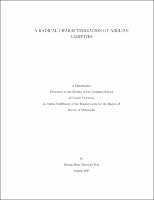## A Radical Characterization of Abelian Varieties##### Abstract
Let $A$ be a square-free abelian variety defined over a number field $K$. Let $S$ be a density one set of prime ideals $\p$ of $\mathcal{O}_K$. A famous theorem of Faltings says that the Frobenius polynomials $P_{A,\p}(x)$ for $\p\in S$ determine $A$ up to isogeny. We show that the prime factors of $|A(\FF_\p)|=P_{A,\p}(1)$ for $\p\in S$ also determine $A$ up to isogeny over an explicit finite extension of $K$. The proof relies on understanding the $\ell$-adic monodromy groups which come from the $\ell$-adic Galois representations of $A$, and the absolute Weyl group action on their weights. We also show that there exists an explicit integer $e\geq 1$ such that after replacing $K$ by a suitable finite extension, the Frobenius polynomials of $A$ at $\p$ must equal to the $e$-th power of a separable polynomial for a density one set of prime ideals $\p\subseteq\mathcal{O}_K$.
2017-08-30
##### Keywords
radical; Galois representations; Mathematics; abelian varieties; monodromy groups
##### Committee Chair
Zywina, David J.
Ramakrishna, Ravi Kumar
Speh, Birgit E M
Mathematics
##### Degree Name
Ph. D., Mathematics
##### Degree Level
Doctor of Philosophy
##### Types
dissertation or thesis